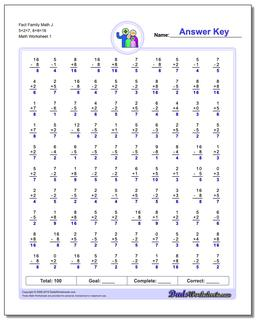# Math Worksheets: Fact Family Math: Fact Family Math: Fact Family Math J 5+2=7, 8+8=16## Fact Family Math J 5+2=7, 8+8=16

PropertyValue
DescriptionFact Family Math J 5+2=7, 8+8=16: These math worksheets have 100 addition and subtraction fact family problems and make for a challenging two minute test.
Resource TypeWorksheet